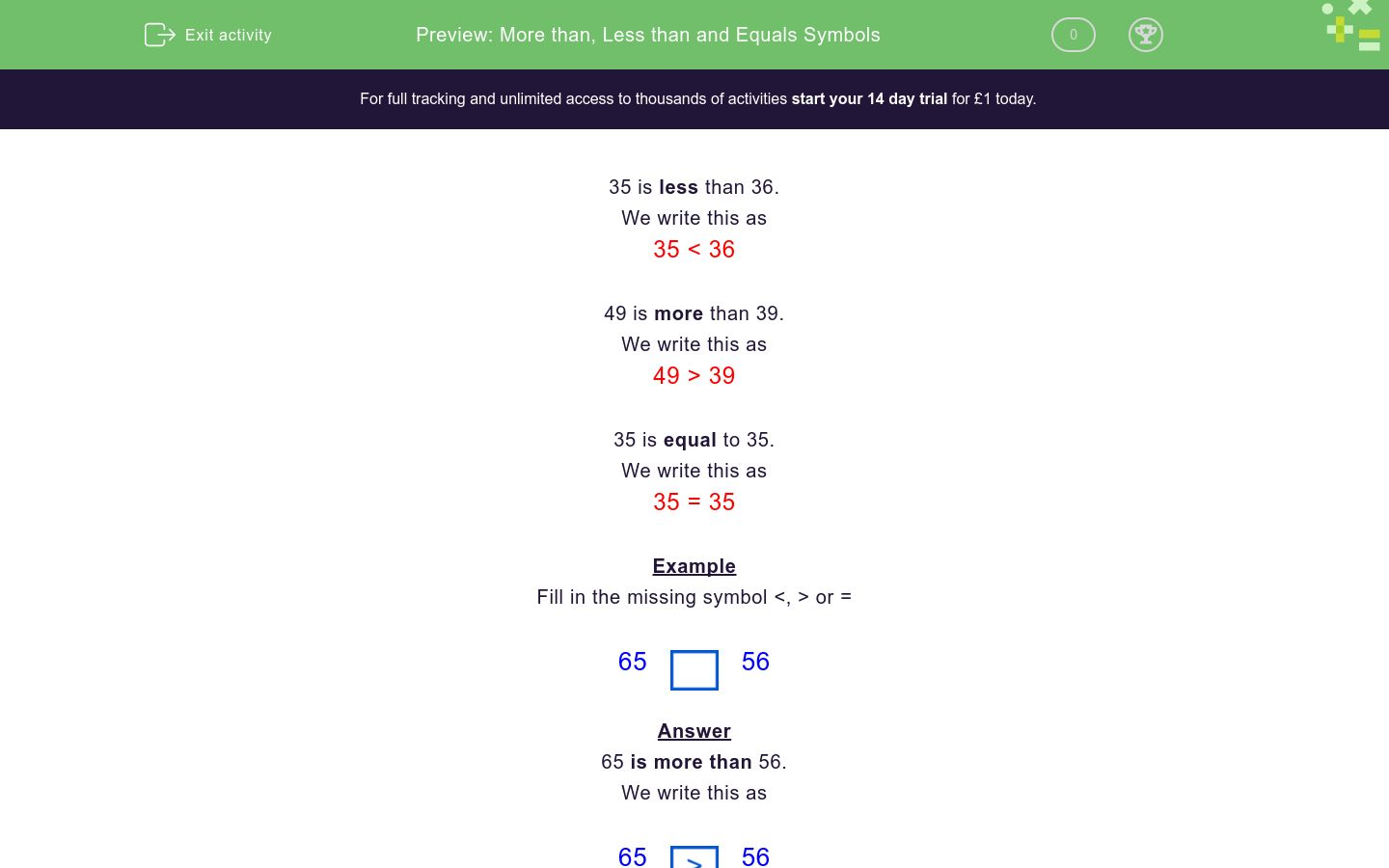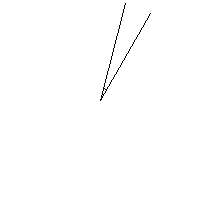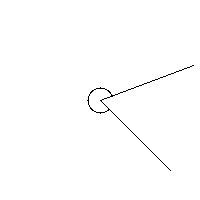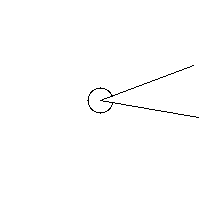# Measuring and Recognising Different Types of Angle

In this worksheet, students measure and recognise different types of angle.Key stage:  KS 3

Curriculum topic:   Geometry and Measures

Curriculum subtopic:   Draw and Measure Line Segments and Angles

Difficulty level:### QUESTION 1 of 10

In this worksheet, you must measure angles and say which type of angle they are.

Example:

Measure these angles, using a protractor.  Say whether they are acute (less than 90°), a right angle (= 90°), obtuse (between 90° and 180°) or reflex (more than 180°).

An angle measures the rotation of one line segment on to another. Angles are measured in degrees, °.AB is turned through 50° to coincide with AC. The angle is labelled BAC.A quarter rotation measures 90° and is marked with a square.A half rotation measures 180°.A complete rotation measures 360°.

Measure this angle using a protractor.

In the first answer box, state whether this angle is acute, obtuse, right angle or reflex.

In the second answer box, state its size in degrees (just write the number).Measure this angle using a protractor.

In the first answer box, state whether this angle is acute, obtuse, right angle or reflex.

In the second answer box, state its size in degrees (just write the number)Measure this angle using a protractor.

In the first answer box, state whether this angle is acute, obtuse, right angle or reflex.

In the second answer box, state its size in degrees (just write the number).Measure this angle using a protractor.

In the first answer box, state whether this angle is acute, obtuse, right angle or reflex.

In the second answer box, state its size in degrees (just write the number).Measure this angle using a protractor.

In the first answer box, state whether this angle is acute, obtuse, right angle or reflex.

In the second answer box, state its size in degrees (just write the number).Measure this angle using a protractor.

In the first answer box, state whether this angle is acute, obtuse, right angle or reflex.

In the second answer box, state its size in degrees (just write the number).Measure this angle using a protractor.

In the first answer box, state whether this angle is acute, obtuse, right angle or reflex.

In the second answer box, state its size in degrees (just write the number).Measure this angle using a protractor.

In the first answer box, state whether this angle is acute, obtuse, right angle or reflex.

In the second answer box, state its size in degrees (just write the number).Measure this angle using a protractor.

In the first answer box, state whether this angle is acute, obtuse, right angle or reflex.

In the second answer box, state its size in degrees (just write the number).Measure this angle using a protractor.

In the first answer box, state whether this angle is acute, obtuse, right angle or reflex.

In the second answer box, state its size in degrees (just write the number).• Question 1

Measure this angle using a protractor.

In the first answer box, state whether this angle is acute, obtuse, right angle or reflex.

In the second answer box, state its size in degrees (just write the number).Reflex
280
• Question 2

Measure this angle using a protractor.

In the first answer box, state whether this angle is acute, obtuse, right angle or reflex.

In the second answer box, state its size in degrees (just write the number)Right angle
90
• Question 3

Measure this angle using a protractor.

In the first answer box, state whether this angle is acute, obtuse, right angle or reflex.

In the second answer box, state its size in degrees (just write the number).Obtuse
155
• Question 4

Measure this angle using a protractor.

In the first answer box, state whether this angle is acute, obtuse, right angle or reflex.

In the second answer box, state its size in degrees (just write the number).Acute
15
• Question 5

Measure this angle using a protractor.

In the first answer box, state whether this angle is acute, obtuse, right angle or reflex.

In the second answer box, state its size in degrees (just write the number).Right angle
90
• Question 6

Measure this angle using a protractor.

In the first answer box, state whether this angle is acute, obtuse, right angle or reflex.

In the second answer box, state its size in degrees (just write the number).Acute
65
• Question 7

Measure this angle using a protractor.

In the first answer box, state whether this angle is acute, obtuse, right angle or reflex.

In the second answer box, state its size in degrees (just write the number).Reflex
295
• Question 8

Measure this angle using a protractor.

In the first answer box, state whether this angle is acute, obtuse, right angle or reflex.

In the second answer box, state its size in degrees (just write the number).Obtuse
175
• Question 9

Measure this angle using a protractor.

In the first answer box, state whether this angle is acute, obtuse, right angle or reflex.

In the second answer box, state its size in degrees (just write the number).Obtuse
105
• Question 10

Measure this angle using a protractor.

In the first answer box, state whether this angle is acute, obtuse, right angle or reflex.

In the second answer box, state its size in degrees (just write the number).Reflex
330
---- OR ----

Sign up for a £1 trial so you can track and measure your child's progress on this activity.

### What is EdPlace?

We're your National Curriculum aligned online education content provider helping each child succeed in English, maths and science from year 1 to GCSE. With an EdPlace account you’ll be able to track and measure progress, helping each child achieve their best. We build confidence and attainment by personalising each child’s learning at a level that suits them.

Get started